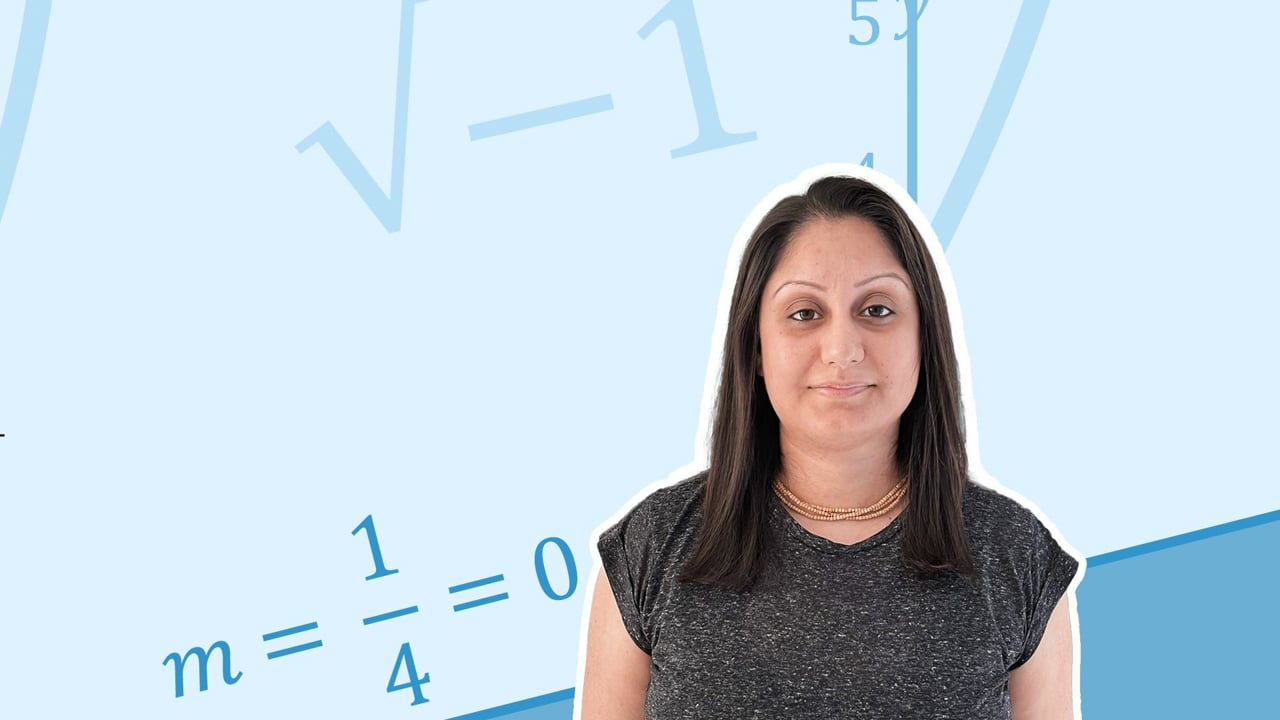Chapter overviewMaths

Types of numbers

Number calculations

Fractions, decimals and percentages

Algebraic manipulation

Formulae and equations

Straight line graphs

Other graphs

Ratio

Proportion

Rates of change

Shapes

Properties of shapes

Measures

Lines and angles

Drawing shapes

Trigonometry

Probability

Statistics

Maths

# Simplifying algebraic expressions0%

Summary

# Simplifying algebraic expressions

## ​​In a nutshell

Algebraic terms which have the same letter are called 'like terms'. Like terms can be added or subtracted, e.g. $2x + 3x=5x$. This is called simplifying. If terms have different letters, they cannot be simplified, e.g. $5x+3y$. Take care with $+$ or $-$signs. The $-$ belongs to the term it is in front of.

##### Example

In the expression $2x-7t$, the $-$ belongs to $7t$.

## Simplifying expressions

Terms with different powers cannot be added or subtracted..

##### Examples
 ​$5a+10b-2a-3b$​​ ​$3a+7b$​​ ​$3x^2+2x$​​ cannot be simplified further ​$3x^2+2x-x^2+7x$​​ ​$2x^2+9x$​​ ​$4xy+3x+xy$​​ ​$3x+5xy$​​

It is possible to use expressions to help you solve geometric problems.

##### Example 1

Write an expression for the perimeter of the following shape.

 ​$m$​​ ​$n$​​Perimeter $=\underline{2m+2n}$

##### Example 2

Write an expression for the perimeter of the following shape.  Simplify your answer as much as possible.

 ​$x+y$​​​$x+y$​​ ​$3x-y$​​

Perimeter $=x+y+x+y+3x-y$

Perimeter $=\underline{5x+2y}$

FAQs

• Question: Can you simplify terms with different letters?

Answer: If terms have different letters, they cannot be simplified. For example, 2a+3b cannot be simplified further.

• Question: How do you simplify expression in algebra?

Answer: You can simplify expressions by adding or subtracting 'like terms'. Like terms contain the same letter or same combination of letters.

• Question: What are 'like terms' in algebra?

Answer: Algebraic terms which have the same letter are called 'like terms'. They can be added or subtracted.

Theory

Exercises